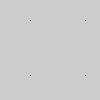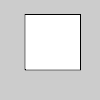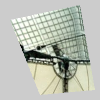# vertex()#

Add a new vertex to a shape.

## Examples#def setup():
py5.begin_shape(py5.POINTS)
py5.vertex(30, 20)
py5.vertex(85, 20)
py5.vertex(85, 75)
py5.vertex(30, 75)
py5.end_shape()def setup():
py5.size(100, 100, py5.P3D)
# drawing vertices in 3D requires P3D
# as a parameter to size()
py5.begin_shape()
py5.vertex(30, 20, 10)
py5.vertex(85, 20, 10)
py5.vertex(85, 75, 10)
py5.vertex(30, 75, 10)
py5.end_shape(py5.CLOSE)def setup():
py5.size(100, 100, py5.P3D)
py5.no_stroke()
py5.begin_shape()
py5.texture(img)
# "laDefense.jpg" is 100x100 pixels in size so
# the values 0 and 100 are used for the
# parameters "u" and "v" to map it directly
# to the vertex points
py5.vertex(10, 20, 0, 0)
py5.vertex(80, 5, 100, 0)
py5.vertex(95, 90, 100, 100)
py5.vertex(40, 95, 0, 100)
py5.end_shape()


## Description#

Add a new vertex to a shape. All shapes are constructed by connecting a series of vertices. The vertex() method is used to specify the vertex coordinates for points, lines, triangles, quads, and polygons. It is used exclusively within the begin_shape() and end_shape() functions.

Drawing a vertex in 3D using the z parameter requires the P3D renderer, as shown in the second example.

This method is also used to map a texture onto geometry. The texture() function declares the texture to apply to the geometry and the u and v coordinates define the mapping of this texture to the form. By default, the coordinates used for u and v are specified in relation to the image’s size in pixels, but this relation can be changed with the Sketch’s texture_mode() method.

Underlying Processing method: vertex

## Signatures#

vertex(
v: npt.NDArray[np.floating],  # vertical coordinate data for the texture mapping
/,
) -> None

vertex(
x: float,  # x-coordinate of the vertex
y: float,  # y-coordinate of the vertex
/,
) -> None

vertex(
x: float,  # x-coordinate of the vertex
y: float,  # y-coordinate of the vertex
u: float,  # horizontal coordinate for the texture mapping
v: float,  # vertical coordinate for the texture mapping
/,
) -> None

vertex(
x: float,  # x-coordinate of the vertex
y: float,  # y-coordinate of the vertex
z: float,  # z-coordinate of the vertex
/,
) -> None

vertex(
x: float,  # x-coordinate of the vertex
y: float,  # y-coordinate of the vertex
z: float,  # z-coordinate of the vertex
u: float,  # horizontal coordinate for the texture mapping
v: float,  # vertical coordinate for the texture mapping
/,
) -> None


Updated on March 06, 2023 02:49:26am UTC